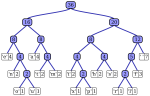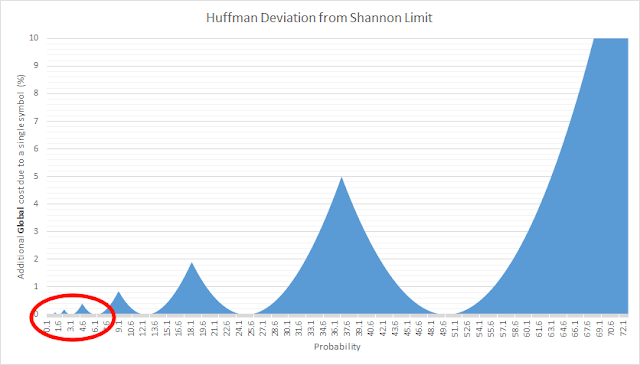#Huffman compression is a well known entropic compression technique since the 1950's. It's optimal, in the sense there is no better construction if one accept the limitation of using an integer number of bits per symbol, a constraint that can severely limit its compression capability in presence of high probability symbols. Huffman compression is very popular, and quite rightly so, thanks to its simplicity and clarity. (It's also patent-free which helps too). For a long time, it remained the entropic compressor of choice due to its excellent speed / efficiency trade off. Today, we can use more powerful entropic compressors such as Arithmetic Coding or the newer ANS based Finite State Entropy, which are able to grab fractional bits, hence ensuring a better compression ratio, closer to the Shannon Limit. The Shannon Limit must be considered like the speed of light, as a hard wall that cannot be crossed. Anytime someone claims the contrary, it is either hiding some cost portions (such as headers, or the decoder itself), or solving a different problem, entangling modeling and entropy. As long as entropy alone is considered, there is simply no way to beat the Shannon Limit. You can just get closer to it. This leads us to a simple question : are there situations where Huffman compression is good enough, meaning that it is so close to Shannon limit that there is very little gain remaining, if any ? The answer to this question is yes. Let's forget some curious corner cases where symbol frequencies are clean power of 2. Of course, in such case, Huffman compression would be optimal, but this is way too specific to consider. Let's therefore imagine a more "natural" situation where all symbol frequencies are randomly scattered along the probability axis, with the sole condition that the sum of all probabilities must be equal to 1. A simple observation : the more numerous the symbols, the most likely each symbol probability is going to be small (since their total sum must be equal to 1). This is an important observation. When the probability of a symbol is small, its deviation from the nearest power of 2 is also small. At some point, this deviation becomes negligible. (Edit : strictly speaking, it's a bit more complex than that. The power of low probability symbols also comes from their combinatorial effects : they help the huffman tree to be more balanced. But that part is more complex to analyze, so just take my word for it.)Therefore, if we are in a situation where no symbol get a large probability (<10%), Huffman compression is likely to provide a "good enough" compression result, meaning close enough to the hard "Shannon limit" so that it doesn't matter to get even closer to it. In a compression algorithm such as Zstandard, the literals are symbols which belong to this category. They are basically the "rest" from LZ compression, which couldn't be identified as part of repeated sequences. They can be any byte value from 0 to 255, which means every symbol get an average of 0.4% probability. Of course, there are some large differences between most common and less common ones, especially on text files. But in practice, most probabilities remain small, so Huffman deviation should be negligible. In Zstandard, all symbols are compressed using Finite State Entropy, which is very fast and performs fractional bit compression. We are saying that, for literals, fractional bit makes little difference, so Huffman can be "good enough". So could we use Huffman instead of FSE for such symbols ? This would only make sense if Huffman compression could bring some kind of advantage on the table, for example speed, and/or memory usage. Alas, currently known versions of Huffman perform worse than Finite State Entropy. The zlib reference version, which is pretty good, max out at 250-300 MB/s, which isn't close to FSE results. My own, older, version of Huffman, huff0, is not even as good as the zlib one. But it's not game over. After all, analysing FSE algorithm in detail, there is no reason for it to be faster than Huffman, since their complexity are similar. A fast, modern, Huffman compressor should reach equivalent speed, if not better on the compression side (due to an additional operation required by FSE to provide fractional bit). Part of the reasons why FSE is fast is that it uses some clever bitStream techniques, combining multiple symbols into branchless writes, a trick which is not strictly tied to FSE and can be used into different context. So the idea was to re-use the bitStream interface, and combine with a Huffman compressor. huff0 was refurbished and improved to employ FSE bitStream. In order to preserve code compatibility, I kept FSE design of compressing and decompressing in reverse directions, which is not strictly necessary for Huffman. I could test though that it does not make any noticeable difference for Huffman compression, making this feature a non-event as long as it remains hidden within block API level. Moving huff0 to this new bitStream proved extremely easy. And the result was very rewarding. With little effort, I could make it reach 500 MB/s compression speed, way better than any other huffman compressor I'm aware of, and more critically way better than FSE compression, making it a replacement candidate. With such great result at hand, I confidently proceeded to implement huffman decompression based on the same design. I was in for a nasty surprise ...

#### 1 comment:

1.I am glad to read this post, its an interesting one. I am always searching for quality posts and articles and this is what I found here, I hope you will be adding more in future. Thanks